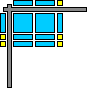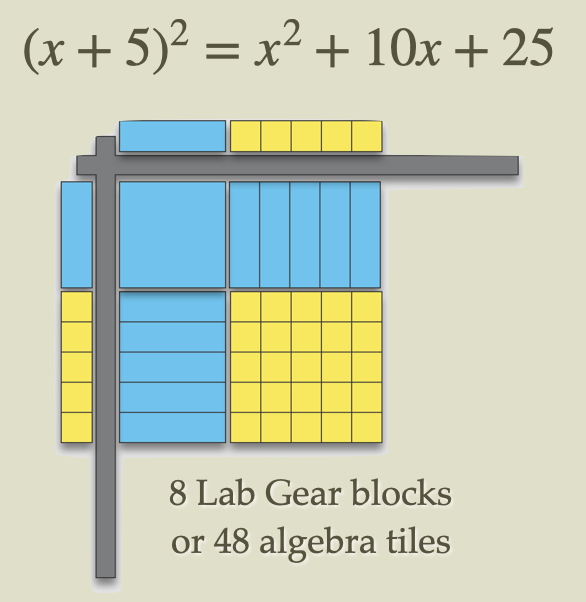# Lab Gear Q and AHenri Picciotto

This page includes answers to many big-picture questions about the Lab Gear, a manipulative environment for the learning of algebra. Just click the triangles to see the answers.
• For a full online training on the Lab Gear, see these videos.
• For links to all the information available on this site, see the Lab Gear Home Page.
• To purchase Lab Gear materials, go to Didax.
Algebra manipulatives? why?
• For many students, manipulating x's and y's on paper does not carry much meaning. They find it hard to get interested in that, and hard to remember algebraic techniques. Manipulatives help give some substance to the subject.
• Having a geometric representation of the concepts gives all students a deeper and more durable understanding.
• Manipulatives provide a change of pace and liven up the algebra classroom.
• Manipulatives facilitate collaboration. Students find it easier to discuss physical models than abstract symbols.
• Manipulatives improve classroom discourse: the teacher talks less, the students talk more.
Blocks vs. tiles: which is better? Tiles are cheaper, but in every other respect blocks are preferable. The Lab Gear model uses blocks for the following reasons:
• Blocks are much easier to manipulate
• Blocks make it possible to explore expressions involving third-degree terms, such as x^3, x^2y, and so on.
• Blocks allow for exploration of surface area.
• The Lab Gear model is an expansion of Base Ten blocks, which many students are already familiar with.
• According to teachers who have used both, blocks are vastly more popular with students.
What are some special features of the Lab Gear?
• Convenience: In addition to the basic 1, x, y, xy, x^2, and y^2 blocks, the Lab Gear includes blocks to represent 5, 25, 5x, and 5y. This makes it possible to quickly represent larger products. Here is an example:Doing this with tiles would take a very long time.
Likewise, representing (x+5)(x+6) would require 11 Lab Gear blocks, or 56 algebra tiles.
• The corner piece: That is the grey item in the illustration. It helps organize the blocks, and provides a place to represent the length and width of the rectangle. (A perfect square, in this case.)
What curricular materials support the Lab Gear? There are two Algebra Lab Gear books: Basic Algebra and Algebra 1. They can be used in conjunction with any algebra or pre-algebra curriculum. They stand out because of these features:
• A puzzle-like approach to many concepts
• Detailed lesson plans, including on how to transition away from reliance on manipulatives
• Connections to graphing
• An enormous range of topics (see below)
What are priority topics with the Lab Gear? At all grade levels:
• The main use of the Lab Gear (and any algebra manipulatives) is to illustrate the distributive property by using the area model for factoring, multiplication, and division.
• In addition, the perimeter and surface area problems help motivate and practice combining like terms, while at the same time making a welcome geometry connection.
Is the Lab Gear useful in middle school? Yes! In addition to the topics mentioned above:
• The Lab Gear provides an approach to integer arithmetic which is compatible with...
• ...the work on linear equations and inequalities.
Does the Lab Gear support more advanced topics? Yes! You can use the Lab Gear for crucial work on quadratics:
• The zero product principle
• Perfect square trinomials
• Completing the square
• Connections with the graphs of parabolas
• Simplifying some algebraic fractions
Is there an overall list of Lab Gear topics? You probably won't have time for all of them, but here you go:
• Integer arithmetic
• Variables and operations
• Simplifying expressions
• The distributive law
• Factoring
• Identities
• Simple polynomial division
• Simple algebraic fractions
• Equations and inequalities
• Simultaneous equations
• Completing the square
• Perimeter, area
• Volume, surface area
• ...and more
The books include Common Core correlations.
Won't it take too much time to add this to my course? It is time well-spent, as the resulting understanding means you can cut back on the amount of practice and review.
• The first year, start with the priority topics listed above. As you and your students get used to the Lab Gear, you can expand beyond those.
• Note that it is not necessary to synchronize this work with the textbook. I recommend front-loading Lab Gear activities — do all you're going to do before winter break, following the logic in the Lab Gear books.
Do you have tips for Lab Gear users? The Lab Gear books start with tips on classroom organization, lab etiquette, sequencing, and so on. Detailed lesson plans for each topic are followed by activities you can duplicate for your class.
• Get plastic boxes from a dollar store — two students per box
• Use felt on desks if the noise bothers you
• Streamline the clean-up routine — it can be done in a couple of minutes
• Allow Lab Gear use in quizzes and tests — the time it takes to get the blocks quickly convinces students to learn how to just work on paper